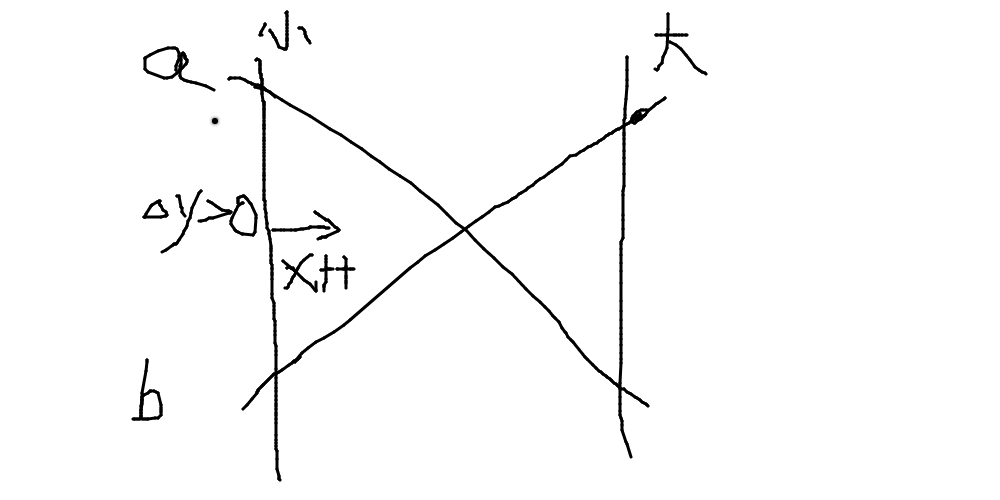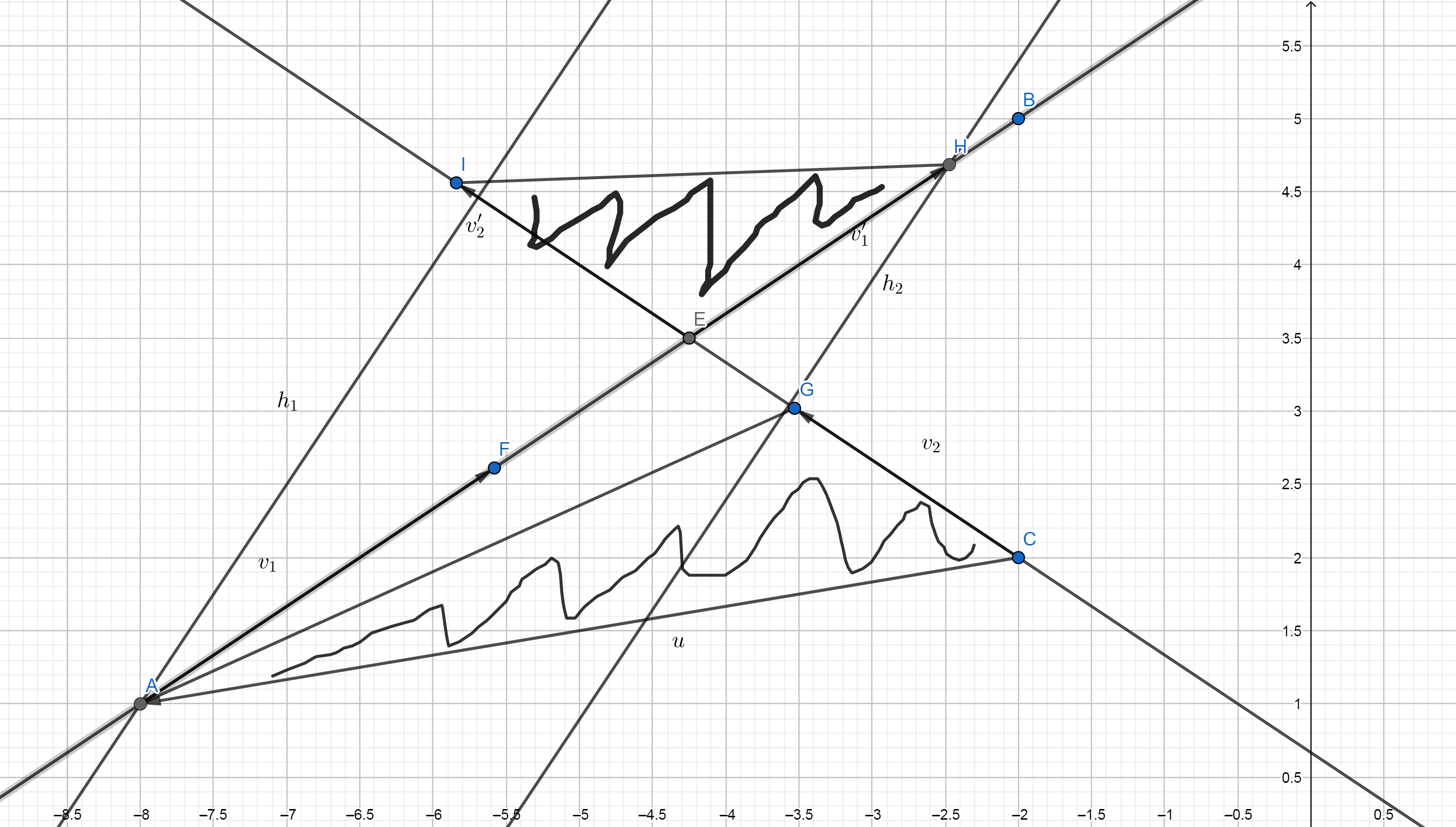• c++一个平面内，两条线段交点坐标。传入四个坐标点，返回一个坐标点。
• 该程序属于MFC编程，应用了基本对话框，实现了画直线等基本操作，并能够交点MFC
• 遂从最简单的直角坐标系两条直线交点开始，直线1的方程解析式: 2x-y=0;直线2的方程解析式: 4x-5y=9;记录下思考过程版本一//直角坐标系中直线2x-y=0　和　4x-5y=9的交点坐标$x = 0;$solution = array();while...

看UdaCity机器学习大纲，发现入门班中有一条：编写算法计算一组直线或平面的交点。

遂从最简单的直角坐标系两条直线的交点开始，

直线1的方程解析式: 2x-y=0;

直线2的方程解析式: 4x-5y=9;

记录下思考过程

版本一

//求直角坐标系中直线2x-y=0　和　4x-5y=9的交点坐标

$x = 0;$solution = array();

while(true){

// 2*$x-$y==0; //这样写不能被程序正确理解

$y = 2*$x; //将2x-y=0转化成这样进行赋值操作

//if(2*$x-$y==0 && 4*$x-5*$y==9){ 这样写不能被程序正确理解

if(4*$x-5*$y==9){ //如果此时的x,y坐标也满足第二个解析式则为解

$solution[] = array('x'=>$x,'y'=>$y); //将坐标计入解集合中 break;//由于两条相交的直线最多只有一个交点，只有一个解，找到这个解后直接跳出循环 } //如果不满足第二个解析式则x变量自减0.1继续下一轮循环$x -= 0.1;

}

var_dump($solution); 结果 /opt/wwwroot/test/test9.php:19: array (size=1) 0 => array (size=2) 'x' => float -1.5 'y' => float -3 问题 1, 可以看到当x=0不符合要求后，x一直在进行自减，并恰好找到解，这是人为干预的巧合．因为仅凭x=0不是解并不能判断x是正数还是负数，也就不能判断x应该进行自减还是自增操作．如果x进行了x+=0.1的自增操作(实际上一般都是自增)，则该循环将一直找不到正解，陷入无限循环． 2, x变量自增减幅度问题．这里得到的解x=-1.5刚好是自变值0.1的整数倍，从而符合条件被找到．如果正解为-1.51，则不会被0.1的自变量命中，它从-1.5和-1.6之间漏过去了，从而也一直找不到这个正解，陷入无限循环．打个比方一批黄金被埋在0-1000米的直路上的某点，某人从0米开始每隔1米挖一个坑，假设黄金被埋在15.1米的地方，而他挖的坑在第15和第16米的地方，则他找不到黄金，即使他每隔0.5米挖一个坑也找不到，除非每隔0.1米挖一个坑，于是他会错过埋在15.01米处的珍珠．这是采样间隔和采样数量的问题． 版本二 为了避免上面的第一个问题，T提出先对x使用一个大小值区间，观察此时的y是如何变化的，再决定对x进行自增还是自减操作//求直角坐标系中直线2x-y=0 和 4x-5y=9的交点坐标 //起始小值$xMin = -10000;

//起始大值

$xMax = 10000; //大小值区间$xZone = $xMax-$xMin;

//解集合

$solution = array(); function line1($x){

$y = 2*$x;

return $y; } function line2($x){

5*$y = 4*$x-9;

return $y; } //返回同一个x值时的y差值(y2-y1) function dY($x){

$y1 = line1($x);

$y2 = line2($x);

return $dY =$y2-$y1; } function getFixPoint($xMin, $xMax){$dYMin = dY($xMin);$dYMax = dY($xMax); //判断体系 //如果两个x最值的y差值相乘为负，则说明y差值一正一负，两线相交于最值区间内，最小值自增，最大值自减，互相逼近 if($dYMin*$dYMax<0){$xMin *= 0.5;

$xMax *= 0.5; getFixPoint($xMin, $xMax); //递归调用本方法 }elseif($dYMin*$dYMax>0){ //如果两个x最值的y差值相乘为正，则说明y差值同号，x的最值区间处于交点的同一侧，需要移动最值区间 if($dYMIn+$dYMax>0){ //如果两个x最值的y差值相加为正，则说明y2>y1,y2在此区间中处于y1上方 //如果y2的斜率大于y1，则交点在区间左边，最值区间往左移，再递归调用本方法 //如果y2的斜率小于y1，则交点在区间右边，最值区间往右移，再递归调用本方法 }elseif($dYMIn+$dYMax<0){ //如果两个x最值的y差值相加为负，则说明y2 //如果y2的斜率大于y1，则交点在区间右边，最值区间往右移，再递归调用本方法 //如果y2的斜率小于y1，则交点在区间左边，最值区间往左移，再递归调用本方法 } }else{ //如果两个y差值相乘为0，则说明两点相交 } } 思路 1, 使用区间的方式来判断交点的位置，避免了很多运算，节约了资源．(参考0-1000猜数字的游戏) 2, 斜率之于直线/一次方程/线性，相当于导数之于曲线/二次方程/非线性，在最值区间互相逼近的过程使用了极限的概念，中值定理(?)，为接下来的二次/三次/高次方程的求解提供了思路． 缺点：逻辑判断稍微复杂. 版本三 //求直角坐标系中直线2x-y=0 和 4x-5y=9的交点坐标 function getFixPoint($a1,$b1,$c1,$a2,$b2,$c2){$x=($b2*$c1 - $b1*$c2)/(-1*$a2*$b1 + $a1*$b2);

$y=($a2*$c1 -$a1*$c2)/($a2*$b1 -$a1*$b2); echo 'x='.$x.'';

echo 'y='.\$y.'';

}

getFixPoint(2,-1,0,4,-5,9);

直接使用高斯消元法，so easy!

x=-1.5

y=-3

思考:

1, 使用算法求数学解时，有公式直接套用公式，站在巨人的肩膀上，比自己造轮子要省时省力高效得多；

2, 算法，机器学习，人工智能这些深入进去之后，还是要用微积分，概率论，线性代数，统计学这些基石．

展开全文java两条直线交点
• 求两条直线交点的正确姿势 在白书上看到的,但没有找到解释,于是记录一下. 如果两条直线是用线上一点+方向向量的方式表示的话,可以这样求直线交点(假定存在): v2 interpoint(Line a,Line b) { v2 u=a.P-b.P; ...

求两条直线交点的正确姿势

• 在白书上看到的,但没有找到解释,于是记录一下.
• 如果两条直线是用线上一点+方向向量的方式表示的话,可以这样求直线交点(假定存在):
v2 interpoint(Line a,Line b)
{
v2 u=a.P-b.P;
double t=(b.v*u)/(a.v*b.v);
return a.P+a.v*t;
}
• 这里 $$P$$ 是直线上一点, $$v$$ 是方向向量, $$*$$ 定义为向量叉乘.
• 怎么理解呢?看这张图:• 叉积是两向量围成三角形面积的两倍,那么 $$t$$ 就是两个三角形面积之比,有一条底边长度相同,为 $$|v_2|$$.那么 $$t$$ 也等于高之比,即图中 $$h_1 : h_2$$ .图中两条垂线是平行的,那么由相似知识可知 $$t=AE : |v_1|$$. 那么交点 $$E$$ 的坐标就可以用上面式子算出了.

转载于:https://www.cnblogs.com/jklover/p/10484313.html

展开全文• 2、设y=ax+b,通过斜率可出a的值，通过坐标可以出b的值，这样线段所在直线个方程式就得到了；3、通过个方程式交点，再判断交点是不是落在线段交点的区域中代码：public static Point ...

1、判断斜率相同不，y2-y1/x2-x1,y4-y3/x4-x3,相同则无交点，不相同说明直线有交点则执行第二步；

2、设y=ax+b,通过斜率可求出a的值，通过坐标可以求出b的值，这样两个线段所在直线的两个方程式就得到了；

3、通过两个方程式求出交点，再判断交点是不是落在两线段交点的区域中

代码：public static Point getCrossPoint(Lseg lsegA,Lseg lsegB){

float x;

float y;

float x1=lsegA.getA().getX();

float y1=lsegA.getA().getY();

float x2=lsegA.getB().getX();

float y2=lsegA.getB().getY();

float x3=lsegB.getA().getX();

float y3=lsegB.getA().getY();

float x4=lsegB.getB().getX();

float y4=lsegB.getB().getY();

float k1=Float.MAX_VALUE;

float k2=Float.MAX_VALUE;

boolean flag1=false;

boolean flag2=false;

if((x1-x2)==0)

flag1=true;

if((x3-x4)==0)

flag2=true;

if(!flag1)

k1=(y1-y2)/(x1-x2);

if(!flag2)

k2=(y3-y4)/(x3-x4);

if(k1==k2)

return null;

if(flag1){

if(flag2)

return null;

x=x1;

if(k2==0){

y=y3;

}else{

y=k2*(x-x4)+y4;

}

}else if(flag2){

x=x3;

if(k1==0){

y=y1;

}else{

y=k1*(x-x2)+y2;

}

}else{

if(k1==0){

y=y1;

x=(y-y4)/k2+x4;

}else if(k2==0){

y=y3;

x=(y-y2)/k1+x2;

}else{

x=(k1*x2-k2*x4+y4-y2)/(k1-k2);

y=k1*(x-x2)+y2;

}

}

if(between(x1,x2,x)&&between(y1,y2,y)&&between(x3,x4,x)&&between(y3,y4,y)){

Point point=new Point();

point.setX(x);

point.setY(y);

if(point.equals(lsegA.getA())||point.equals(lsegA.getB()))

return null;

return point;

}else{

return null;

}

}

public static boolean between(float a,float b,float target){

if(target>=a-0.01&&target<=b+0.01||target<=a+0.01&&target>=b-0.01)

return true;

else

return false;

}}

展开全文• #include#define ll long longusing namespace std;struct Point{ll x,y;Point(ll x=0,ll y=0):x(x),y(y){};};ll gcd(ll a,ll b){return a==0?b:gcd(b%a,a);}bool cheak(ll op1,ll a,ll b){if(a>...

#include

#define ll long long

using namespace std;

struct Point{

ll x,y;

Point(ll x=0,ll y=0):x(x),y(y){};

};

ll gcd(ll a,ll b)

{

return a==0?b:gcd(b%a,a);

}

bool cheak(ll op1,ll a,ll b){

if(a>b)

swap(a,b);

return op1>=a&&op1<=b;

}

Point point_of_intersection(Point f1,Point f2,Point f3,Point f4,bool &mark)

{

ll a1,a2,b1,b2,c1,c2,c3,c4,D,D1,D2;

a1=f2.y-f1.y;

a2=f1.x-f2.x;

b1=a1*f1.x+a2*f1.y;

///b1=(y2-y1)*x1+(x1-x2)*y1

c1=f4.y-f3.y;

c2=f3.x-f4.x;

b2=c1*f3.x+c2*f3.y;

///b2=(y4-y3)*x3+(x3-x4)*y3

c3=f2.x-f1.x;

c4=f4.x-f3.x;

D=c3*c1-c4*a1;

Point res;

if(D==0){

mark=false;

return res;

}

D1=b2*c3-b1*c4;

if(D1%D){

mark=false;

return res;

}

res.x=int(D1/D);

D2=b2*a1-b1*c1;

if(D2%D){

mark=false;

return res;

}

res.y=int(D2/D);

if(!cheak(res.x,f1.x,f2.x)||!cheak(res.x,f3.x,f4.x)){

mark=false; return res;

}

if(!cheak(res.y,f1.y,f2.y)||!cheak(res.y,f3.y,f4.y)){

mark=false; return res;

}

return res;

}

Point edge;

int main()

{

int n;

while( ~scanf("%d",&n)){

for(int i=1;i<=n;i++){

scanf("%lld%lld%lld%lld",&edge[i].x,&edge[i].y,&edge[i].x,&edge[i].y);

}

set > re;

long long ans=0,tmp;

bool mark;

for(int i=1;i<=n;i++){

tmp=gcd(abs(edge[i].x-edge[i].x),abs(edge[i].y-edge[i].y))+1;

re.clear();

for(int j=1;j

mark=true;

Point res=point_of_intersection(edge[i],edge[i],edge[j],edge[j],mark);

if(mark)

re.insert(make_pair(res.x,res.y));

}

ans+=tmp-re.size();

}

printf("%lld\n",ans);

}

return 0;

}

展开全文• 设已知的一条线段的起点...首先两条线段的一次函数，再联立交点坐标交点公式： x=(y3x4x2-y4x3x2-y3x4x1+y4x3x1-y1x2x4+y2x1x4+y1x2x3-y2x1x3)/x4y2-x4y1-x3y2+x3y1-x2y4+x2y3+x1y4-x1y3y=(-y3x4y2+y4x3y2+y3算法
• 这个方法转载自评论区 ... # 取直线坐标点的x和y值 x1=line1 y1=line1 x2=line1 y2=line1 x3=line2 y3=line2 x4=line2 y4=line2 # L2直线斜率不存在操作 if (x4-x3)==0: k2=N
• 空间两直线交点-MATLAB代码+原理说明 原理.pdf —— 说明性文档 main_demo.m —— demo主程序，也就是一个示例 Intersection_of_TwoLines.m —— 交点的函数MATLAB 几何
• const double EPS = 1e-5; // 计算精度const int CROSS = 0; // 两直线相交const int COLINE = 1; // ...
• opencv3找直线并任意两条直线交点，并显示出来，是直线交点，因为我把线段延长了。 交点函数 /*函数功能：求两条直线交点*/ /*输入：两条Vec4i类型直线*/ /*返回：Point2f类型的点*/ Point2f getCrossPoint...
• 下面是修正后的/// /// 计算两条直线交点/// /// L1的点1坐标/// L1的点2坐标/// L2的点1坐标/// L2的点2坐标/// public static PointF GetIntersection(PointF lineFirstStar, PointF lineFirstEnd, PointF ...
• 使用PCL库中的直线拟合方法，可以拟合出来多条直线，可以对这多条直线求交点（前提是直线相交）。 对应实现代码如下： PS：如果是多条直线，要首先判断哪些直线的相交的，哪些直线是平行的。要有这个过程。 .....
• python求两条线段的交点

千次阅读 2018-09-25 20:25:34
本来想找一段求线段交点的代码，但是网上很多的代码都没有考虑除0的特殊情况，所以自己写了一份。具体原理： 一般方程法： 直线的一般方程为F(x) = ax + by + c = 0。既然我们已经知道直线个点，假设为(x0,y0)...
• 也可以理解为 ：三角形一个... /// 计算两条直线交点 /// </summary> /// <param name="lineFirstStar">L1的点1坐标</param> /// <param name="lineFirstEnd">L1的点2坐标</param&g...
• 算法，求两直线交点

热门讨论 2011-12-07 12:20:52
• C++球两直线交点MyPoint setpoint(Line &l,Line &m) { MyPoint p; p.x=(m.b-l.b)/(l.a-m.a); p.y=(l.a*m.b-m.a*l.b)/(l.a-m.a); return p; } 这的MyPoint p; 要加个参数 而且不能直接用p.x p.y求两直线交点C++
• JAVA 获取两条直线的交点

千次阅读 2020-07-03 14:47:10
JAVA 获取两条直线交点 代码如下： public class PointIntersect { public static class Point{ private double x; private double y; public Point(double x,double y){ this.x=x; this.y=y; } public...java
• opencv求任意两条直线交点

千次阅读 2018-07-10 12:59:50
函数功能：求两条直线交点 求两条直线交点 /*函数功能：求两条直线交点*/ /*输入：两条Vec4i类型直线*/ /*返回：Point2f类型的点*/ Point2f getCrossPoint(Vec4i LineA, Vec4i LineB) { double ka, kb; ka = ...
• 此函数使用行列式的方法来找到两条直线交点。 该函数的输入是两条线（向量）的坐标，即 Line1 = [(x11,y11);(x12,y12)] 和 line2=[(x21,y21);(x22,y22)]。matlab
• 算法：两条线段求交点

千次阅读 2018-08-20 17:33:00
验证两条线段是否相交时，需求两条线段交点 算法详解： 已知两个线段分别是(x1,y1)(x2,y2)以及(x3,y3)(x4,y4)，假设这两条线段不平行。 设线段所在的直线为ax+by=c a1=y2-y1, b1=-(x2-x1), c1=a1*x1-b1*y1=x1*...
• 如何计算两条线的交点？

千次阅读 2021-02-04 05:06:00
对于DL1 L1 L2 L2 对于DxL1 L1 L2 L2 对于DyL1 L1 L2 L2 现在开始编码： line-通过两点产生线方程的coefsA，B，C， intersection-查找coefs提供的两条线的交点（如果有）。...
• 记录：python 求两直线交点

千次阅读 2020-07-17 17:24:15
本人数学渣渣，公式来自知乎大佬，大佬真是太强了。 ...用方程法，避开了算K，真是太强了x2。 def calc_abc_from_line_2d(x0, y0, x1, y1): a = y0 - y1 b = x1 - x0 c = x0*y1 - x1*y0 return a, b, c ...python 求两直线交点
• 问题描述问题描述：已知两条线段P1P2和Q1Q2，判断P1P2和Q1Q2是否相交，若相交，交点两条线段的位置关系可以分为三类：有重合部分、无重合部分但有交点、无交点。算法核心算法的步骤如下：1.快速排斥实验。设以...
• def point(x0,y0,x1,y1,x2,y2,x3,y3): a = y1-y0 b = x1*y0-x0*y1 c = x1-x0 d = y3-y2 e = x3*y2-x2*y3 f = x3-x2 y = float(a*e-b*d)/(a*f-c*d) x = float(y*c-b)/apython
• Opencv学习笔记-----求取两条直线的交点坐标

万次阅读 多人点赞 2016-12-15 12:06:07
取二直线交点(基于OpenCv) 理论补充： 两直线是否有交点(或线段齐延长线是否相交)是一个Line-line intersection(Wikipedia)问题 在一个2维平面中有两直线(点到点、(点到点，这两条直线的交点用行列式表示如下：...opencv 两直线交点
• 两直线相交 function [X,Y]=pll(X1,Y1,X2,Y2) % 直线相交求交点 A1=Y1(1)-Y1(2); B1=X1(2)-X1(1); C1=Y1(2)*X1(1)-Y1(1)*X1(2); A2...... Matlab与通信仿真主讲教师:和煦 通信基础实验教学中心 第2-1页 内容提要 1 ......